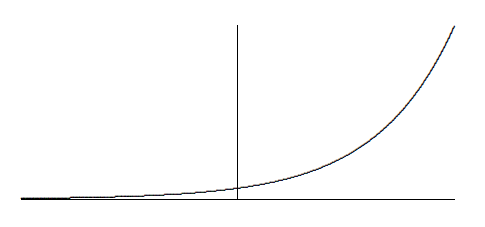$$\newcommand{\id}{\mathrm{id}}$$ $$\newcommand{\Span}{\mathrm{span}}$$ $$\newcommand{\kernel}{\mathrm{null}\,}$$ $$\newcommand{\range}{\mathrm{range}\,}$$ $$\newcommand{\RealPart}{\mathrm{Re}}$$ $$\newcommand{\ImaginaryPart}{\mathrm{Im}}$$ $$\newcommand{\Argument}{\mathrm{Arg}}$$ $$\newcommand{\norm}{\| #1 \|}$$ $$\newcommand{\inner}{\langle #1, #2 \rangle}$$ $$\newcommand{\Span}{\mathrm{span}}$$

# 4.6: Exponential and Logarithmic Functions

•• Contributed by David Guichard
• Professor (Mathematics) at Whitman College
$$\newcommand{\vecs}{\overset { \rightharpoonup} {\mathbf{#1}} }$$ $$\newcommand{\vecd}{\overset{-\!-\!\rightharpoonup}{\vphantom{a}\smash {#1}}}$$$$\newcommand{\id}{\mathrm{id}}$$ $$\newcommand{\Span}{\mathrm{span}}$$ $$\newcommand{\kernel}{\mathrm{null}\,}$$ $$\newcommand{\range}{\mathrm{range}\,}$$ $$\newcommand{\RealPart}{\mathrm{Re}}$$ $$\newcommand{\ImaginaryPart}{\mathrm{Im}}$$ $$\newcommand{\Argument}{\mathrm{Arg}}$$ $$\newcommand{\norm}{\| #1 \|}$$ $$\newcommand{\inner}{\langle #1, #2 \rangle}$$ $$\newcommand{\Span}{\mathrm{span}}$$ $$\newcommand{\id}{\mathrm{id}}$$ $$\newcommand{\Span}{\mathrm{span}}$$ $$\newcommand{\kernel}{\mathrm{null}\,}$$ $$\newcommand{\range}{\mathrm{range}\,}$$ $$\newcommand{\RealPart}{\mathrm{Re}}$$ $$\newcommand{\ImaginaryPart}{\mathrm{Im}}$$ $$\newcommand{\Argument}{\mathrm{Arg}}$$ $$\newcommand{\norm}{\| #1 \|}$$ $$\newcommand{\inner}{\langle #1, #2 \rangle}$$ $$\newcommand{\Span}{\mathrm{span}}$$

An exponential function has the form $$a^x$$, where $$a$$ is a constant; examples are $$2^x$$, $$10^x$$, $$e^x$$. The logarithmic functions are the inverses of the exponential functions, that is, functions that "undo'' the exponential functions, just as, for example, the cube root function "undoes'' the cube function: $$\root3\of{2^3}=2$$. Note that the original function also undoes the inverse function: $$(\root3\of{8})^3=8$$.

Let $$f(x)=2^x$$. The inverse of this function is called the logarithm base 2, denoted $$\log_2(x)$$ or (especially in computer science circles) $$\lg(x)$$. What does this really mean? The logarithm must undo the action of the exponential function, so for example it must be that $$\lg(2^3)=3$$---starting with 3, the exponential function produces $$2^3=8$$, and the logarithm of 8 must get us back to 3. A little thought shows that it is not a coincidence that $$\log(2^3)$$ simply gives the exponent---the exponent is the original value that we must get back to. In other words, the logarithm is the exponent. Remember this catchphrase, and what it means, and you won't go wrong. (You do have to remember what it means. Like any good mnemonic, "the logarithm is the exponent'' leaves out a lot of detail, like "Which exponent?'' and "Exponent of what?'')

Example 4.6.1

What is the value of $$\log_{10}(1000)$$?

Solution

The "10'' tells us the appropriate number to use for the base of the exponential function. The logarithm is the exponent, so the question is, what exponent $$E$$ makes $$10^E=1000$$? If we can find such an $$E$$, then $$\log_{10}(1000)=\log_{10}(10^E)=E$$; finding the appropriate exponent is the same as finding the logarithm. In this case, of course, it is easy: $$E=3$$ so $$\log_{10}(1000)=3$$.

Let's review some laws of exponents and logarithms; let $$a$$ be a positive number. Since

$a^5=a\cdot a\cdot a\cdot a\cdot a$

and

$a^3=a\cdot a\cdot a,$

it's clear that

$a^5\cdot a^3 = a\cdot a\cdot a\cdot a\cdot a\cdot a\cdot a\cdot a = a^8 = a^{5+3},$

and in general that

$a^ma^n=a^{m+n}.$

Since "the logarithm is the exponent,'' it's no surprise that this translates directly into a fact about the logarithm function. Here are three facts from the example: $$\log_a(a^5)=5$$, $$\log_a(a^3)=3$$, $$\log_a(a^8)=8$$. So $$\log_a(a^5a^3)=\log_a(a^8)=8 = 5+3=\log_a(a^5)+\log_a(a^3)$$. Now let's make this a bit more general. Suppose $$A$$ and $$B$$ are two numbers, $$A=a^x$$, and $$B=a^y$$. Then $$\log_a(AB)=\log_a(a^xa^y)=\log_a(a^{x+y})=x+y=\log_a(A)+\log_a(B)$$.

Now consider $$(a^5)^3=a^5\cdot a^5\cdot a^5=a^{5+5+5}=a^{5\cdot 3}=a^{15}$$. Again it's clear that more generally $$(a^m)^n=a^{mn}$$, and again this gives us a fact about logarithms. If $$A=a^x$$ then $$A^y=(a^x)^y=a^{xy}$$, so $$\log_a(A^y)=xy=y\log_a(A)$$---the exponent can be "pulled out in front.''

We have cheated a bit in the previous two paragraphs. It is obvious that $$a^5=a\cdot a\cdot a\cdot a\cdot a$$ and $$a^3=a\cdot a\cdot a$$ and that the rest of the example follows; likewise for the second example. But when we consider an exponential function $$a^x$$ we can't be limited to substituting integers for $$x$$. What does $$a^{2.5}$$ or $$a^{-1.3}$$ or $$a^\pi$$ mean? And is it really true that $$a^{2.5}a^{-1.3}=a^{2.5-1.3}$$? The answer to the first question is actually quite difficult, so we will evade it; the answer to the second question is "yes.''

We'll evade the full answer to the hard question, but we have to know something about exponential functions. You need first to understand that since it's not "obvious'' what $$2^x$$ should mean, we are really free to make it mean whatever we want, so long as we keep the behavior that is obvious, namely, when $$x$$ is a positive integer. What else do we want to be true about $$2^x$$? We want the properties of the previous two paragraphs to be true for all exponents: $$2^x2^y=2^{x+y}$$ and $$(2^x)^y=2^{xy}$$.

After the positive integers, the next easiest number to understand is 0: $$2^0=1$$. You have presumably learned this fact in the past; why is it true? It is true precisely because we want $$2^a2^b=2^{a+b}$$ to be true about the function $$2^x$$. We need it to be true that $$2^02^x=2^{0+x}=2^x$$, and this only works if $$2^0=1$$. The same argument implies that $$a^0=1$$ for any $$a$$.

The next easiest set of numbers to understand is the negative integers: for example, $$2^{-3}=1/2^3$$. We know that whatever $$2^{-3}$$ means it must be that $$2^{-3}2^{3}=2^{-3+3}=2^0=1$$, which means that $$2^{-3}$$ must be $$1/2^3$$. In fact, by the same argument, once we know what $$2^x$$ means for some value of $$x$$, $$2^{-x}$$ must be $$1/2^{x}$$ and more generally $$a^{-x}=1/a^x$$.

Next, consider an exponent $$1/q$$, where $$q$$ is a positive integer. We want it to be true that $$(2^x)^y=2^{xy}$$, so $$(2^{1/q})^q=2$$. This means that $$2^{1/q}$$ is a $$q$$-th root of 2, $$2^{1/q}=\root q\of{2 }$$. This is all we need to understand that

$2^{p/q}=(2^{1/q})^p=(\root q\of{2 })^p$

and

$a^{p/q}=(a^{1/q})^p=(\root q\of{a })^p.$

What's left is the hard part: what does $$2^x$$ mean when $$x$$ cannot be written as a fraction, like $$x=\sqrt{2 }$$ or $$x=\pi$$? What we know so far is how to assign meaning to $$2^x$$ whenever $$x=p/q$$; if we were to graph this we'd see something like this:But this is a poor picture, because you can't see that the "curve'' is really a whole lot of individual points, above the rational numbers on the $$x$$-axis. There are really a lot of "holes'' in the curve, above $$x=\pi$$, for example. But (this is the hard part) it is possible to prove that the holes can be "filled in'', and that the resulting function, called $$2^x$$, really does have the properties we want, namely that $$2^x2^y=2^{x+y}$$ and $$(2^x)^y=2^{xy}$$.